# Quotient

Determine the quotient (q) and the remainder (r) from division numbers 100 and 8. Take the test of correctness.

Result

q =  12
r =  4

#### Solution:Leave us a comment of example and its solution (i.e. if it is still somewhat unclear...):

Showing 0 comments:Be the first to comment!## Next similar examples:

1. Like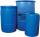When is in 14 barrels 140 liters of water, how many liters barrels of 8 liters I need to get all the water from the larger barrels?
2. DivisionWhich number in division 16 give 12 and the rest 3?
3. Double 5Peter was thinking of a number. Peter doubles it and gets an answer of 8.6. What was the original number?
4. The resultHow many times I decrease the number 1632 to get the result 24?
5. Crates 2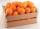One crate will hold 50 oranges. If Bob needs to ship 932 oranges, how many crates will he need?
6. Math classificationIn 3A class are 27 students. One-third got a B in math and the rest got A. How many students received a B in math?
7. DoctorsIn the city operates 171 doctors. The city has 128934 citizens. How many citizens are per one doctor?
8. Wallpaper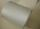Salesman account for 2.3 meters of wallpaper 149.50 Kc. How much cost 1 meter of wallpaper?
9. Cheaper cars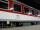State-owned railway company buys 10 air-conditioned coaches for nearly 18 million euros. Calculate how many euros is the equivalent of one seat in the wagon with a capacity of 83 people. How many of used cars at the price 2700 Euros can be buy instead o
10. ProductResult of the product of the numbers 1, 2, 3, 1, 2, 0 is:
11. Roman numeralsWrite numbers written in Roman numerals as decimal.
12. To thousands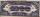Round to thousands following numbers:
13. Valid numberRound the 453874528 on 2 significant numbers.
14. Addition of Roman numbersAdded together and write as decimal number: LXVII + MLXIV
15. Write decimalsWrite in the decimal system the short and advanced form of these numbers: a) four thousand seventy-nine b) five hundred and one thousand six hundred and ten c) nine million twenty-six
16. Unknown number 16My number's tens is three times more then ones My number's ones is twice the number of thousands and my number's hundreds is half the number of of tens. I have two ones. which number am I?
17. Value of teeth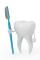To know value to the healthy teeth we discover only when we take care about tooth caries followed by loss of teeth and think how to replace missing teeth... Calculate the value (cost of money) healthy teeth, if we assume that man has with 32 teeth and rep